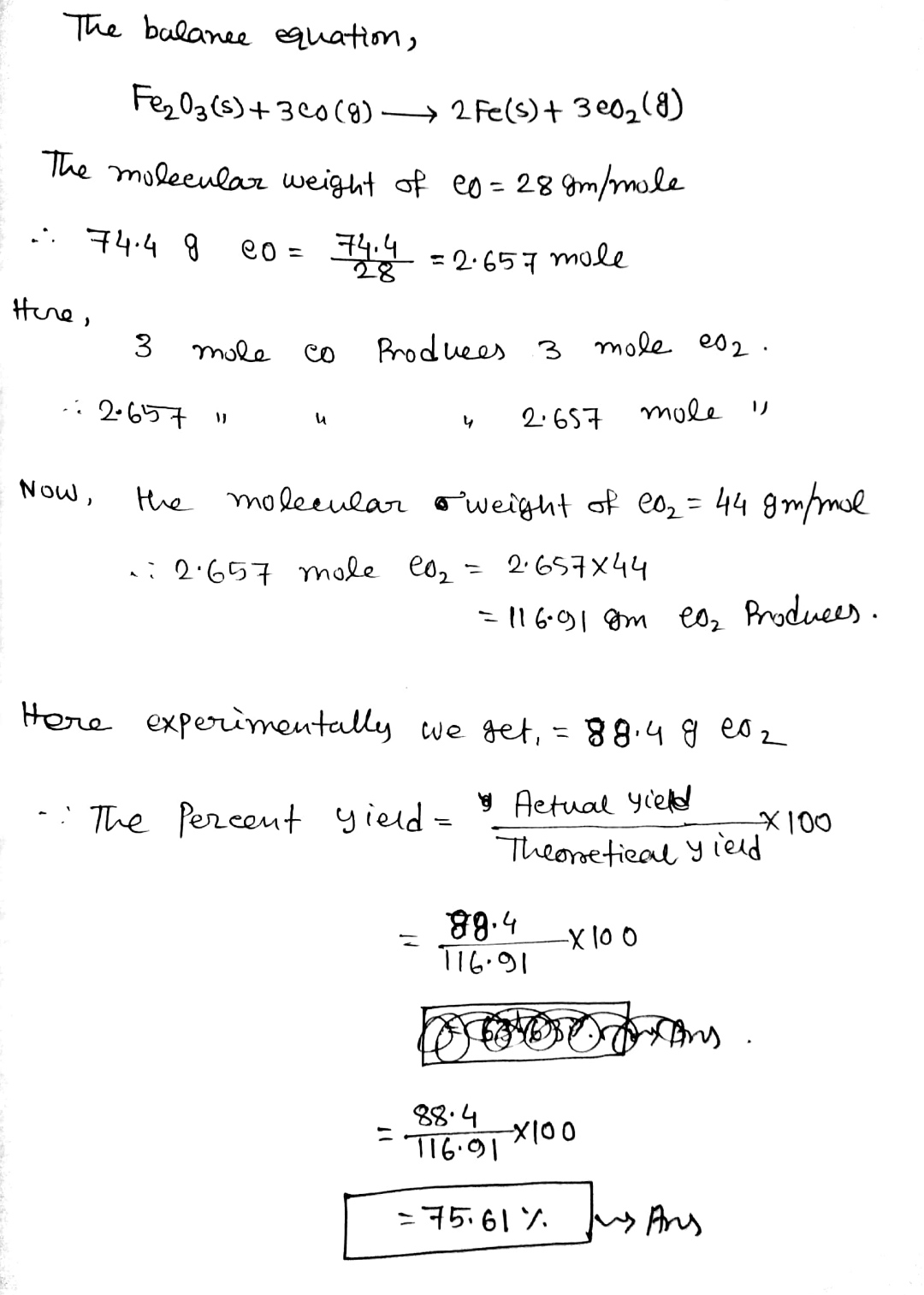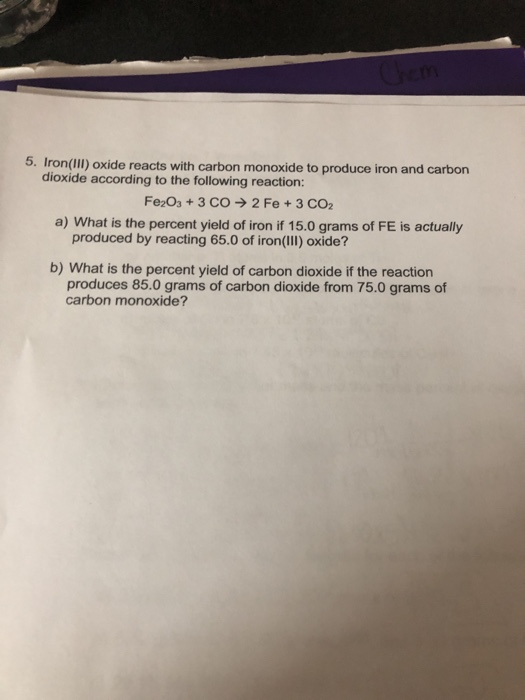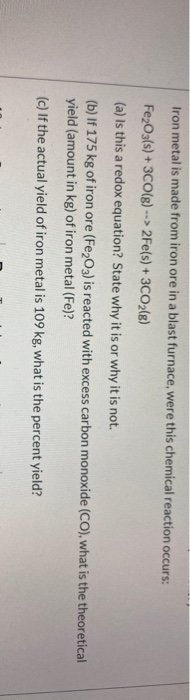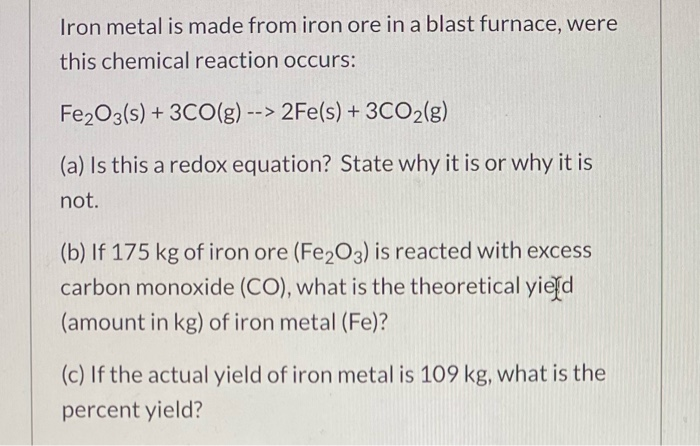Question

What is the percent yield of carbon dioxide if the reaction of 74.4 g of carbon monoxide produces 88.4 g of carbon dioxide?

Iron(III)(III) oxide reacts with carbon monoxide to produce iron and carbon dioxide.

Fe2O3(s)+3CO(g)→2Fe(s)+3CO2(g)#### Earn Coins

Coins can be redeemed for fabulous gifts.

Similar Homework Help Questions
• ### When 50.1 g iron(III) oxide reacts with carbon monoxide, 31.7 g iron is produced. What is...

When 50.1 g iron(III) oxide reacts with carbon monoxide, 31.7 g iron is produced. What is the percent yield of the reaction? Fe2O3(s)+3CO(g)→2Fe(s)+3CO2(g)

• ### Iron(III) oxide reacts with carbon monoxide according to the equation: Fe2O3(s)+3CO(g)→2Fe(s)+3CO2(g)Fe2O3(s)+3CO(g)→2Fe(s)+3CO2(g) A reaction mixture initially contains...

Iron(III) oxide reacts with carbon monoxide according to the equation: Fe2O3(s)+3CO(g)→2Fe(s)+3CO2(g)Fe2O3(s)+3CO(g)→2Fe(s)+3CO2(g) A reaction mixture initially contains 22.85 gg Fe2O3Fe2O3 and 14.26 gg COCO. Once the reaction has occurred as completely as possible, what mass (in gg) of the excess reactant is left? m= ____ g

• ### Iron(III) oxide reacts with carbon monoxide according to the equation: Fe2O3(s)+3CO(g)→2Fe(s)+3CO2(g) A reaction mixture initially contains...

Iron(III) oxide reacts with carbon monoxide according to the equation: Fe2O3(s)+3CO(g)→2Fe(s)+3CO2(g) A reaction mixture initially contains 22.90 g Fe2O3 and 15.90 g CO. Once the reaction has occurred as completely as possible, what mass (in g) of the excess reactant is left?

• ### 5. Iron(III) oxide reacts with carbon monoxide to produce iron and carbon dioxide according to the...5. Iron(III) oxide reacts with carbon monoxide to produce iron and carbon dioxide according to the following reaction: Fe2O3 + 3 CO → 2 Fe + 3 CO2 a) What is the percent yield of iron if 15.0 grams of FE is actually produced by reacting 65.0 of iron(III) oxide? b) What is the percent yield of carbon dioxide if the reaction produces 85.0 grams of carbon dioxide from 75.0 grams of carbon monoxide?

• ### Iron(III) oxide reacts with carbon to give iron and carbon monoxide. Fe2O3(s)+3C(s)→2Fe(s)+3CO(g) How many grams of...

Iron(III) oxide reacts with carbon to give iron and carbon monoxide. Fe2O3(s)+3C(s)→2Fe(s)+3CO(g) How many grams of C are required to react with 18.1 g of Fe2O3?

• ### Iron(III) oxide reacts with carbon to give iron and carbon monoxide. Fe2O3(s)+3C(s)→2Fe(s)+3CO(g) How many grams of...

Iron(III) oxide reacts with carbon to give iron and carbon monoxide. Fe2O3(s)+3C(s)→2Fe(s)+3CO(g) How many grams of C are required to react with 33.5 g of Fe2O3? How many grams of CO are produced when 41.0 g of C reacts?

• ### Blast furnaces extract pure iron from the iron(III) oxide in iron ore in a two step...

Blast furnaces extract pure iron from the iron(III) oxide in iron ore in a two step sequence. In the first step, carbon and oxygen react to form carbon monoxide: 2C (s) + O2 (g) → 2CO (g) In the second step, iron(III) oxide and carbon monoxide react to form iron and carbon dioxide: Fe2O3 (s) + 3CO (g) → 2Fe (s) + 3CO2 (g) Write the net chemical equation for the production of iron from carbon, oxygen and iron(III) oxide....

• ### In a blast furnace, Iron (III) oxide reacts with coke (carbon) to produce molten iron and...

In a blast furnace, Iron (III) oxide reacts with coke (carbon) to produce molten iron and carbon monoxide. Fe2O3 + 3C = 2Fe + 3CO. How many kg of iron would be formed from 125 kg of Fe2O3?

• ### Iron metal is made from iron ore in a blast furnace, were this chemical reaction occurs:...Iron metal is made from iron ore in a blast furnace, were this chemical reaction occurs: Fe2O3(s) + 3CO(g) --> 2Fe(s) + 3CO2(g) (a) Is this a redox equation? State why it is or why it is not. (b) If 175 kg of iron ore (Fe2O3) is reacted with excess carbon monoxide (CO), what is the theoretical yield (amount in kg) of iron metal (Fe)? (c) If the actual yield of iron metal is 109 kg, what is the percent...

• ### Iron metal is made from iron ore in a blast furnace, were this chemical reaction occurs:...Iron metal is made from iron ore in a blast furnace, were this chemical reaction occurs: Fe2O3(s) + 3CO(g) --> 2Fe(s) + 3CO2(g) (a) Is this a redox equation? State why it is or why it is not. (b) If 175 kg of iron ore (Fe2O3) is reacted with excess carbon monoxide (CO), what is the theoretical yield (amount in kg) of iron metal (Fe)? (c) If the actual yield of iron metal is 109 kg, what is the percent...